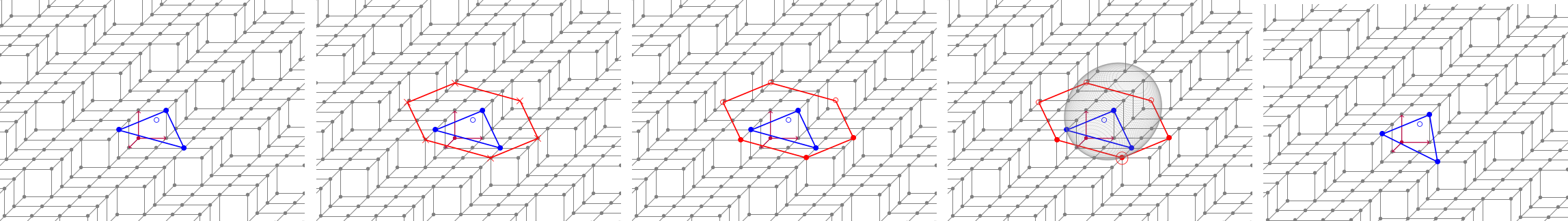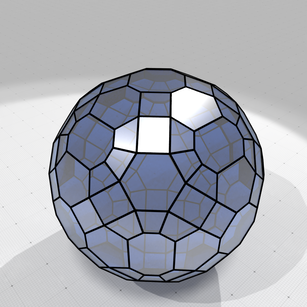We are really excited to share with you the release 1.2 of DGtal and its tools. As usual, all edits and bugfixes are listed in the Changelog, and we would like to thank all devs involved in this release. In this short review, we would like to focus on only few of them.

## Python binding

Yes.. it has been quite a long requested feature and we are pleased to introduce you the brand new (partial) python binding (kudos Pablo Hernandez-Cerdan). At this point, only a few collections of classes/functions are available in the binding (mostly, basic kernels, some image containers and many topology classes), but everything is set up for further improvements.

To give you a glimpse, just

``````pip3 install dgtal
``````

and then here you have just a quick example of the computation of the Euler characteristic and simple point tests on random sets:

``````import dgtal
import random

Point  = dgtal.kernel.Point3D
Domain = dgtal.kernel.DomainZ3i
Set    = dgtal._dgtal.kernel.DigitalSetZ3i

dom    = Domain( Point(0,0,0), Point(10,10,10))
mySet  = Set(dom)

# Random set
for i in range(50*50):
mySet.insert(Point(random.randint(0,10),random.randint(0,10),random.randint(0,10)))

# Digital Object (with topology)
Object = dgtal.topology.Object26_6
Topo   = Object.TDigitalTopology
obj    = Object(topo,mySet)

# Checking the simple points
cptSimple=0
for p in mySet:
if obj.isSimple(p):
cptSimple += 1
print("Number of simple points: "+str(cptSimple)+ " / " + str(mySet.size()))

# Cubical Complex
kspace   = dgtal.topology.KSpace3D()
ccomplex = dgtal.topology.CubicalComplex3D(kspace)
ccomplex.construct(mySet)

print("Euler characteristic: "+str(ccomplex.euler()))
``````

To learn more about the binding, please check this Pull-Request. Please note that all python objects have a pretty self-exploratory documentation. Just use `ipython` to inspect the objects.

## Geometry

Many new features on the Geometry package, including plane probing based normal vector estimators, and the Quickhull algorithm in arbitrary dimensional lattice space, used to speed-up the new convexity package that was introduced in the release 1.1.